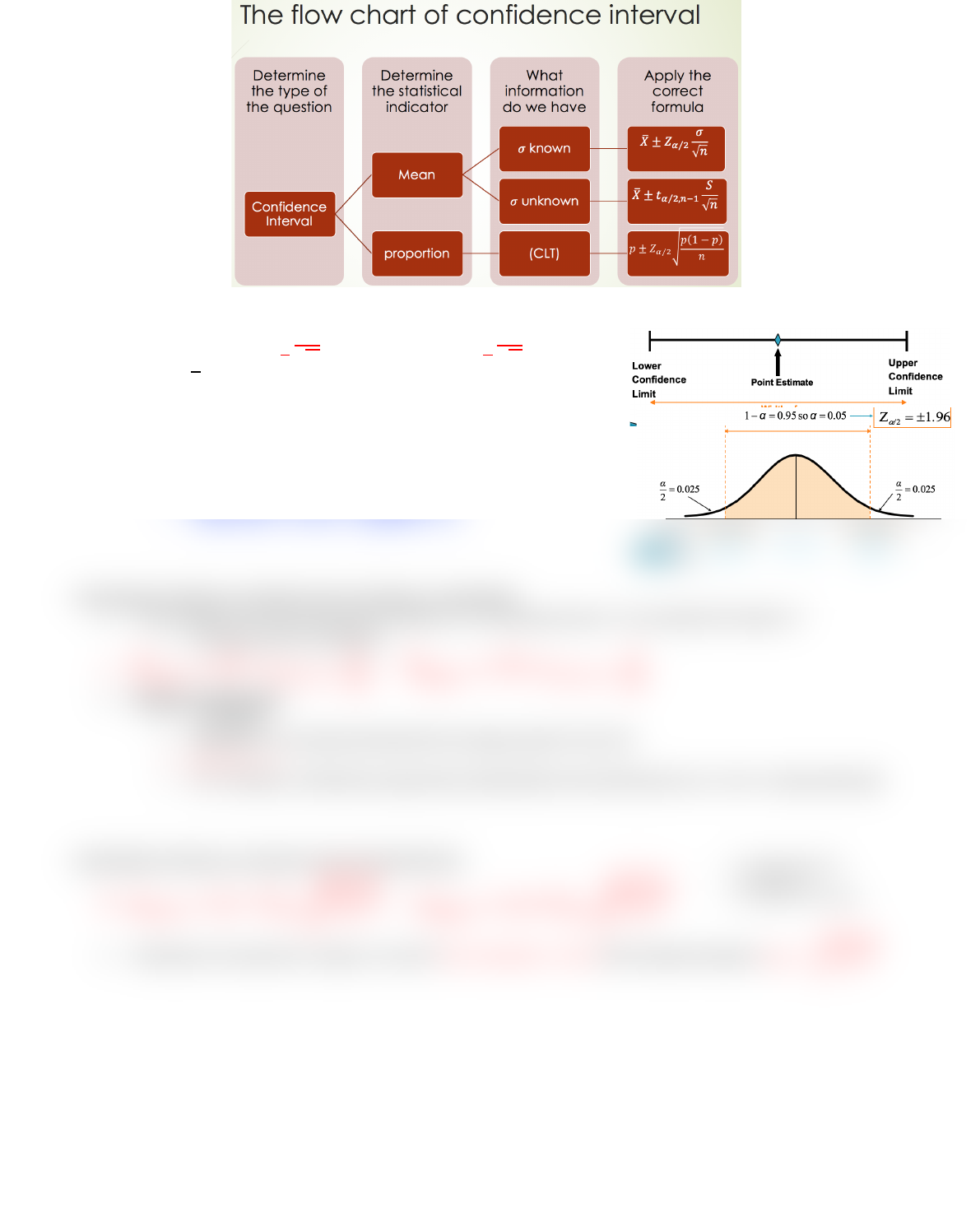# BUSS1020 Chapter Notes - Chapter 8: Confidence Interval, Standard Deviation, Point Estimation

39 views2 pages
School
Course
ProfessorCHAPTER 8: CONFIDENCE INTERVALS
Confidence interval: used to determine the percentage of sample means within certain distances of pop mean
o Confidence level (1-α): confidence that interval will contain the unknown pop parameter
o
!"#\$%
&'%#()%&
±
*+#%#*),
-),.&
× (
'%)\$/)+/
&++"+
)
CONFIDENCE INTERVAL ESTIMATE FOR THE MEAN (σ KNOWN)
0
1
23456 7 0
1
89:
;<
=
>
?
@0
1
ABB56 7 0
1
C9:
;<
=
>
?
o
D?
= point estimate
o Zα/2 = critical value
Assumptions:
o Population standard deviation is known
o Population is normally distributed OR use large sample (CLT)
Finding critical value Zα/2
o 95% confidence interval à Confidence level = 1 α = 0.05
o In table find 0.975 (0.05) à Zα/2 value = 1.96
CONFIDENCE INTERVALL ESTIMATE FOR THE MEAN (σ UNKNOWN)
In the majority of real world business situations, σ is not exactly known à use sample SD instead = S
o Introduces more uncertainty
0
1
23456 7 0
1
8%E F@>GH
I
J
=
>@
?????
0
1
ABB56 7 0
1
C%E F@>GH
I
J
=
>
Student-t distribution:
o σ unknown
o Population is normally distributed OR use large sample if not (CLT)
o
/KLK7\$8M
o As n increases, t distribution approaches standardized normal distribution (n>= 120 = virtually identical)
CONFIDENCE INTERVALL ESTIMATE FOR THE PROPORTION
N23456 7N89E F
IO
B
P
HGB
Q
>@
?????
NABB56 7 NC9E F
IO
B
P
HGB
Q
>
Distribution of proportion is approx. normal if
\$R
≥ 5
)\$/
\$
(1
R
) 5 with standard deviation
SB7
O
T
P
HGT
Q
>
- p = sample proportion
- n = sample size
- π = population proportion
Unlock document

This preview shows half of the first page of the document.
Unlock all 2 pages and 3 million more documents.

## Get access

\$8 USD/m\$10 USD/m
Billed \$96 USD annuallyHomework Help
Study Guides
Textbook Solutions
Class Notes
Textbook Notes
Booster Class